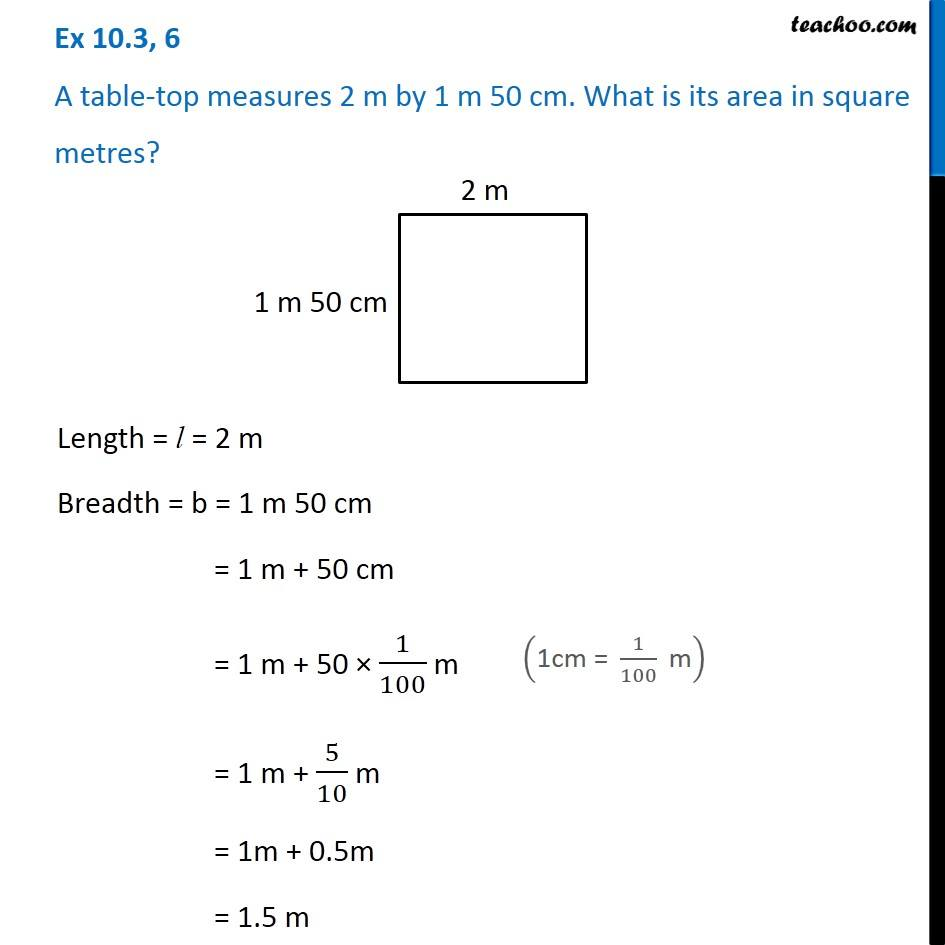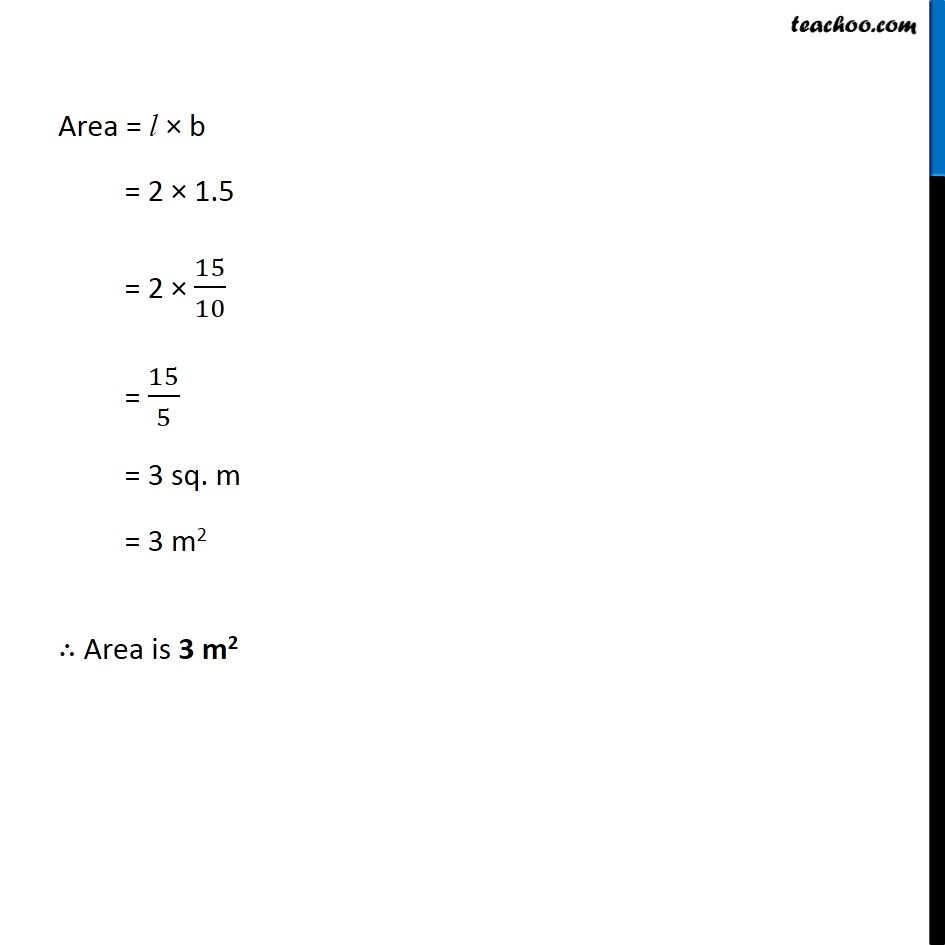Ex 10.3

Chapter 10 Class 6 Mensuration
Serial order wiseGet live Maths 1-on-1 Classs - Class 6 to 12

### Transcript

Ex 10.3, 6 A table-top measures 2 m by 1 m 50 cm. What is its area in square metres? Length = l = 2 m Breadth = b = 1 m 50 cm = 1 m + 50 cm = 1 m + 50 × 1/100 m = 1 m + 5/10 m = 1m + 0.5m = 1.5 m ("1cm = " 1/100 " m" ) Area = l × b = 2 × 1.5 = 2 × 15/10 = 15/5 = 3 sq. m = 3 m2 ∴ Area is 3 m2